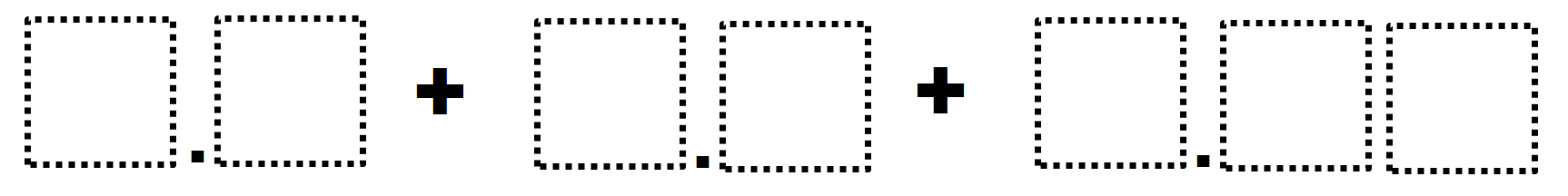Directions: Use the digits 0 to 9, at most one time each, to fill in the boxes so that the sum is as close to 10 as possible.### Hint

How can we get close to 10 by adding digits in the ones place?

8.2 + 1.3 + 0.49
8.3 + 1.2 + 0.49
8.2 + 1.4 + 0.29
8.3 + 1.4 + 0.29

Source: Giselle Garcia

## Solving One-Step Inequalities with Addition

Directions: Using the digits 0 to 9 at most one time each, place a digit …

1.•Sorry, I’m not seeing it. What part needs to be edited?

•I don’t know what to do here anyways.

2.The directions say only digits 1 to 9. Please correct if you are using the digit zero (0). My solution was 5.6 + 1.4 + 2.98 using your allowed digits.

•Good catch. I’ll fix it.

3.5.7+1.8+2.49

My 6th graders said you could not use 0 in the directions 🙂

•Good catch. I’ll fix it.

4.Here is the beauty of Open – middle: discussions about what you didn’t think about.

I think in the original problem you could replace the box for the numbers place with a zero.

OR you could allow zero to be used, then?
5.9 + 2.3 + 1.80 !
Technically my answer is incorrect because the original problem says “”…as close to…” not to actually reach 10, so: 6.9 + 2.3 + 0.81 ! (You didn’t say we couldn’t go over 10)

I’m sure there is more!

•Totally Franco. Lots of possibilities.

5.Such a great problem for even your 7th and 8th graders. The decision for a student to work the problems by stacking and adding as rearrangement is essential the flexible math thinking. So many students do not go there until it is brought up in discussion or presented as another student’s work.

6.In your hint, do you mean, “How can we get close to ten by adding digits in the ones place?”

•Yes. Thank you for catching this error, which has now been fixed.

7.So, do the directions specifically imply that the sum cannot actually BE 10?
If not, 5.9 + 2.3 + 1.80 – thanks to my student Jennifer M.

I have another answer that equals 9.99- thanks to my student Anthony B
6.8 + 2.4 + 0.79

8.Eso es el mimo dijito porque quiere decir que es el mismo dijito

9.what do you do? its to confusing

10.4.6+3.5+1.27=9.37

11.I cant even use the picture if just shows a photo can you put a text box on the boxes so we can write?

12.The boxes just come as a picture can you put text boxes so we can type our answers ?

13.One of my students did 4.7+3.5+1.80

14.1.8+3.2+5.4=10.4

15.I got 10.27 by adding 4.1+3.5+2.67 sigh almost got it 🙁

16.1: 7.6+2.3+0.14 = 10.04
2: 6.5+3.4+0.12 = 10.02

17.One of my students, Sophia, found another solution that gives a sum of exactly 10!
4.8 + 3.7 + 1.50 = 10

18.I got 4.1 + 3.0 + 2.89=9.99

19.Bryce Bowlin 1st pd

5.7+2.1+2.1=9.9

20.Our class came up with
5.9 + 2.4 + 1.70 = 10
4.5 + 3.6 + 1.90= 10

21.8.4+1.3+.29